# presentation.pptx

29 de May de 2023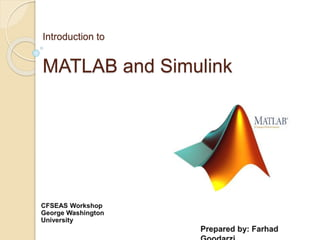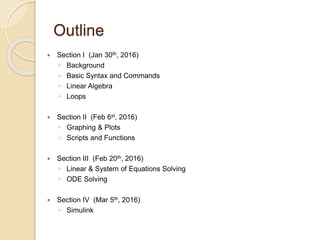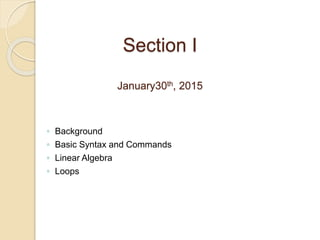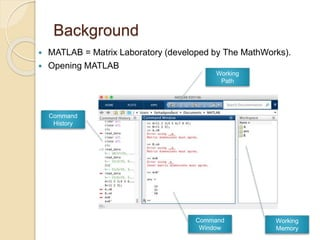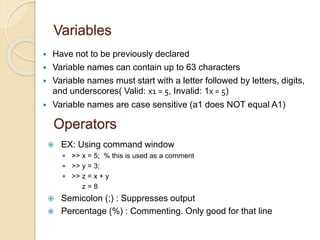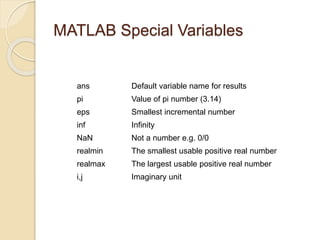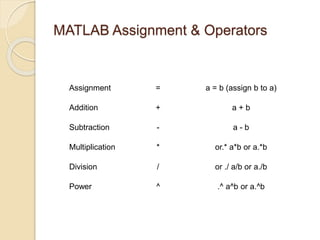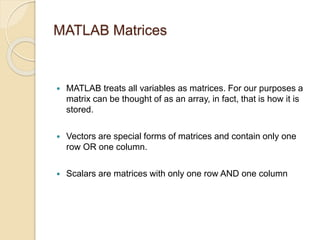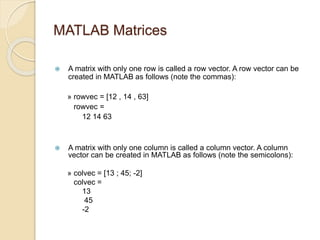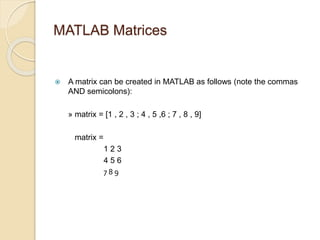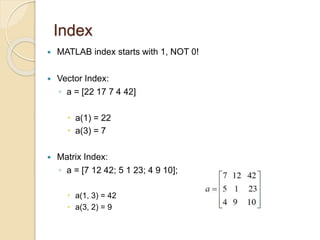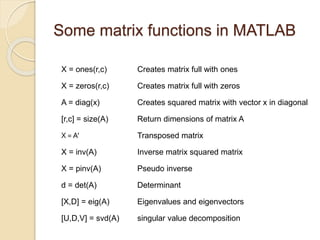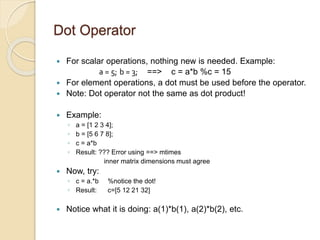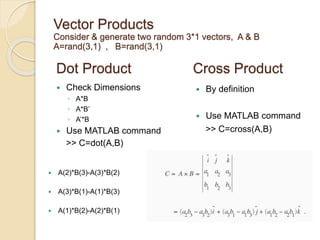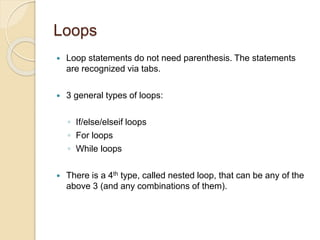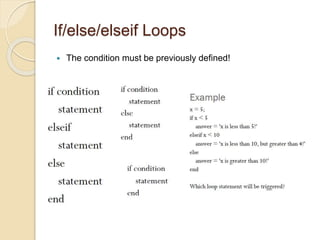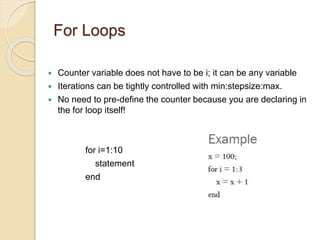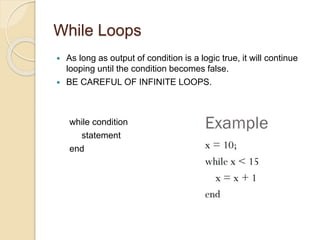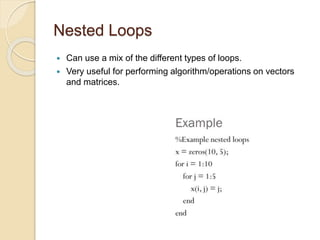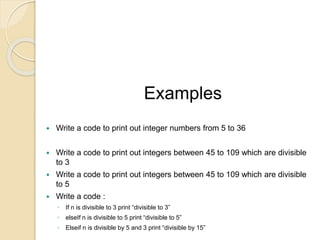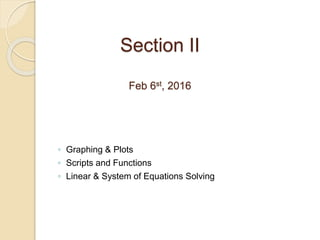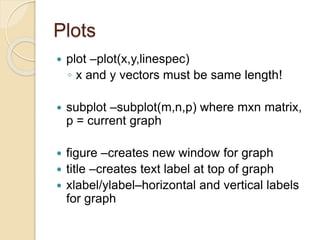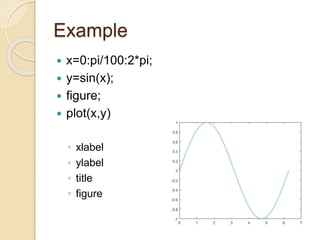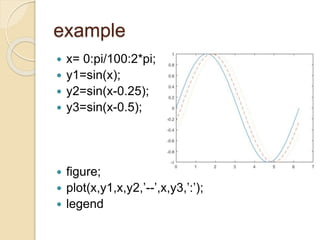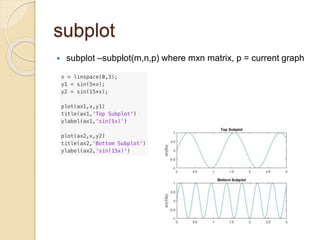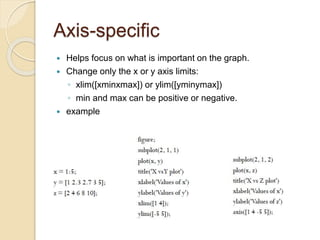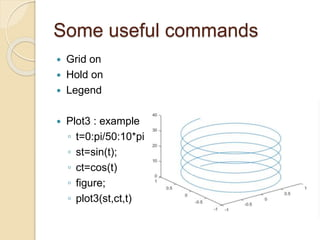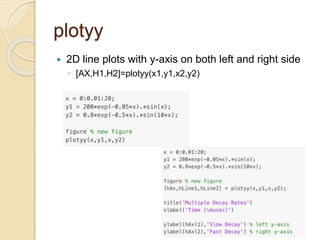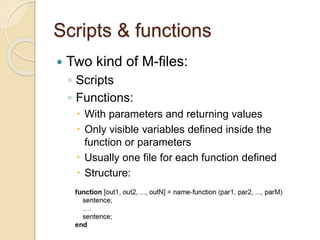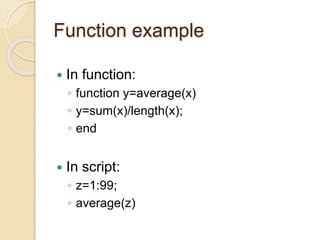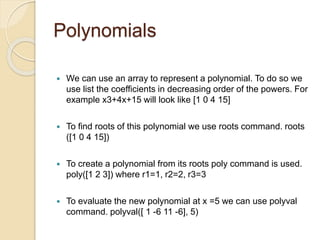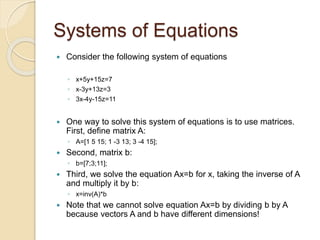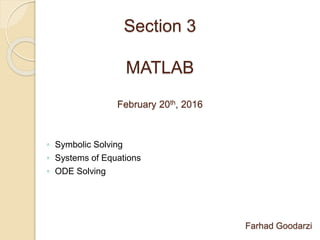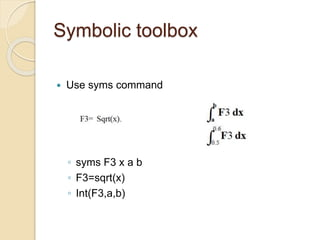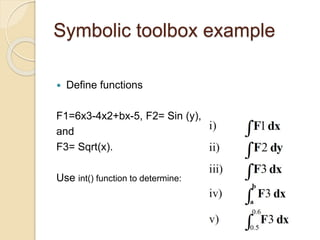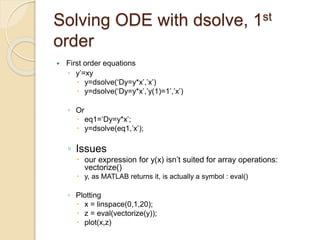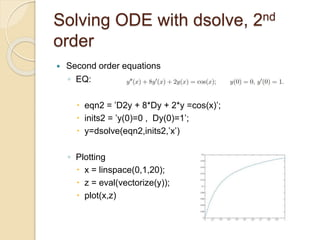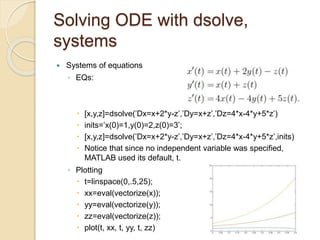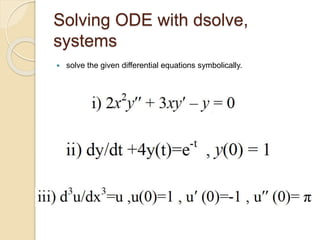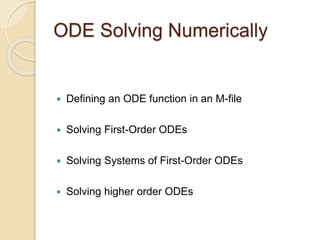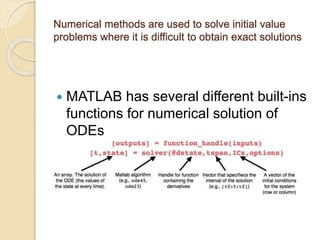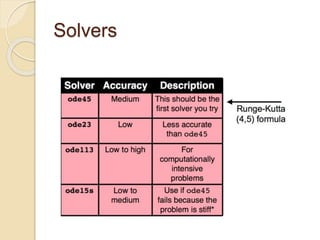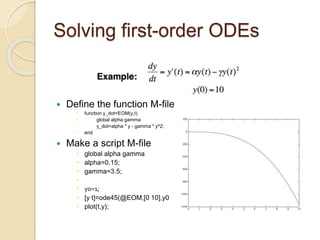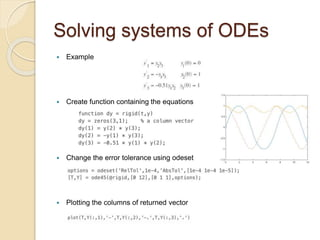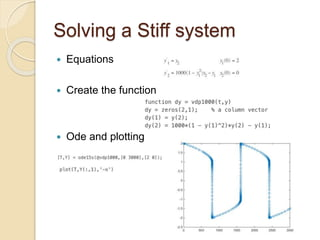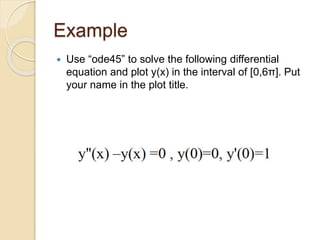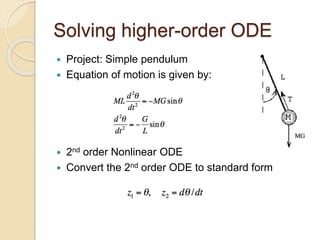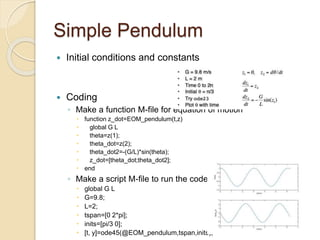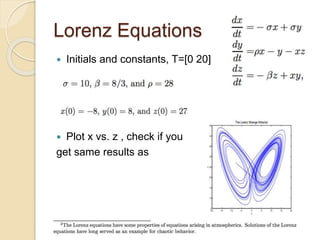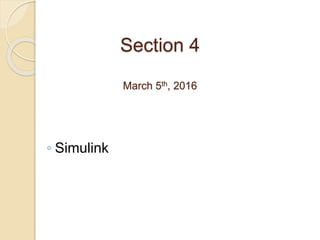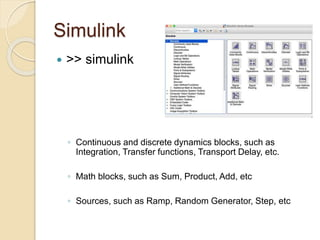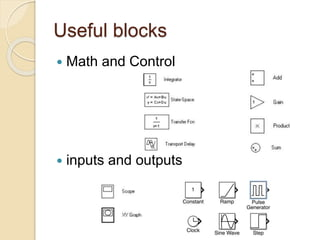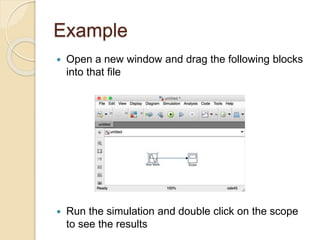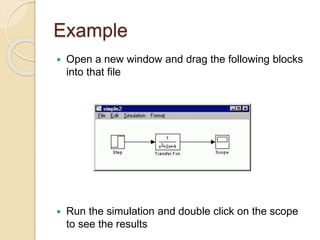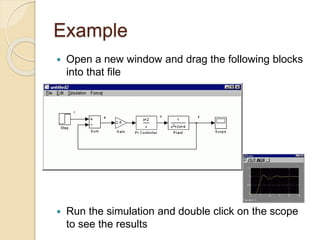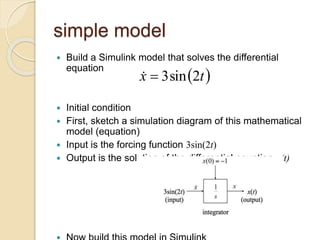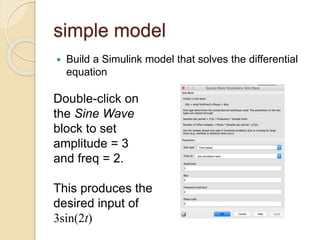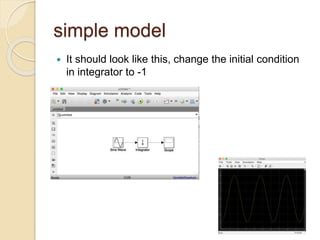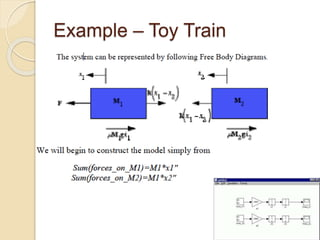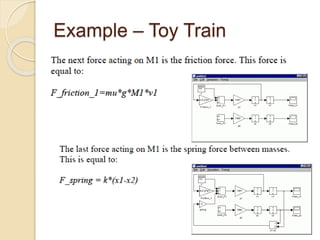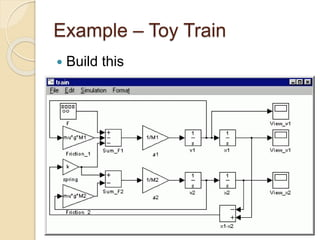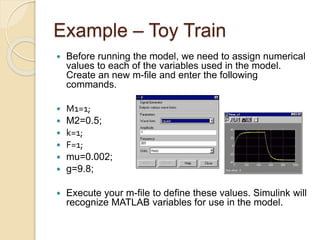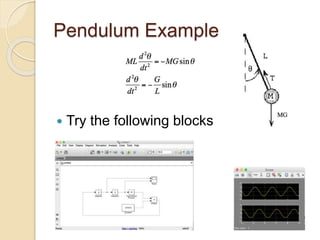1 de 65

### presentation.pptx

• 2. Outline  Section I (Jan 30th, 2016) ◦ Background ◦ Basic Syntax and Commands ◦ Linear Algebra ◦ Loops  Section II (Feb 6st, 2016) ◦ Graphing & Plots ◦ Scripts and Functions  Section III (Feb 20th, 2016) ◦ Linear & System of Equations Solving ◦ ODE Solving  Section IV (Mar 5th, 2016) ◦ Simulink
• 3. Section I January30th, 2015 ◦ Background ◦ Basic Syntax and Commands ◦ Linear Algebra ◦ Loops
• 4. Background  MATLAB = Matrix Laboratory (developed by The MathWorks).  Opening MATLAB Working Memory Command History Command Window Working Path
• 5. Variables  Have not to be previously declared  Variable names can contain up to 63 characters  Variable names must start with a letter followed by letters, digits, and underscores( Valid: x1 = 5, Invalid: 1x = 5)  Variable names are case sensitive (a1 does NOT equal A1)  EX: Using command window  >> x = 5; % this is used as a comment  >> y = 3;  >> z = x + y z = 8  Semicolon (;) : Suppresses output  Percentage (%) : Commenting. Only good for that line Operators
• 6. MATLAB Special Variables ans Default variable name for results pi Value of pi number (3.14) eps Smallest incremental number inf Infinity NaN Not a number e.g. 0/0 realmin The smallest usable positive real number realmax The largest usable positive real number i,j Imaginary unit
• 7. MATLAB Assignment & Operators Assignment = a = b (assign b to a) Addition + a + b Subtraction - a - b Multiplication * or.* a*b or a.*b Division / or ./ a/b or a./b Power ^ .^ a^b or a.^b
• 8. MATLAB Matrices  MATLAB treats all variables as matrices. For our purposes a matrix can be thought of as an array, in fact, that is how it is stored.  Vectors are special forms of matrices and contain only one row OR one column.  Scalars are matrices with only one row AND one column
• 9. MATLAB Matrices  A matrix with only one row is called a row vector. A row vector can be created in MATLAB as follows (note the commas): » rowvec = [12 , 14 , 63] rowvec = 12 14 63  A matrix with only one column is called a column vector. A column vector can be created in MATLAB as follows (note the semicolons): » colvec = [13 ; 45; -2] colvec = 13 45 -2
• 10. MATLAB Matrices  A matrix can be created in MATLAB as follows (note the commas AND semicolons): » matrix = [1 , 2 , 3 ; 4 , 5 ,6 ; 7 , 8 , 9] matrix = 1 2 3 4 5 6 7 8 9
• 11. Index  MATLAB index starts with 1, NOT 0!  Vector Index: ◦ a = [22 17 7 4 42]  a(1) = 22  a(3) = 7  Matrix Index: ◦ a = [7 12 42; 5 1 23; 4 9 10];  a(1, 3) = 42  a(3, 2) = 9
• 12. Some matrix functions in MATLAB X = ones(r,c) Creates matrix full with ones X = zeros(r,c) Creates matrix full with zeros A = diag(x) Creates squared matrix with vector x in diagonal [r,c] = size(A) Return dimensions of matrix A X = A’ Transposed matrix X = inv(A) Inverse matrix squared matrix X = pinv(A) Pseudo inverse d = det(A) Determinant [X,D] = eig(A) Eigenvalues and eigenvectors [U,D,V] = svd(A) singular value decomposition
• 13. Dot Operator  For scalar operations, nothing new is needed. Example: a = 5; b = 3; ==> c = a*b %c = 15  For element operations, a dot must be used before the operator.  Note: Dot operator not the same as dot product!  Example: ◦ a = [1 2 3 4]; ◦ b = [5 6 7 8]; ◦ c = a*b ◦ Result: ??? Error using ==> mtimes inner matrix dimensions must agree  Now, try: ◦ c = a.*b %notice the dot! ◦ Result: c=[5 12 21 32]  Notice what it is doing: a(1)*b(1), a(2)*b(2), etc.
• 14. Dot Product  Check Dimensions ◦ A*B ◦ A*B’ ◦ A’*B  Use MATLAB command >> C=dot(A,B) Cross Product  By definition  Use MATLAB command >> C=cross(A,B) Vector Products Consider & generate two random 3*1 vectors, A & B A=rand(3,1) , B=rand(3,1)  A(2)*B(3)-A(3)*B(2)  A(3)*B(1)-A(1)*B(3)  A(1)*B(2)-A(2)*B(1)
• 15. Loops  Loop statements do not need parenthesis. The statements are recognized via tabs.  3 general types of loops: ◦ If/else/elseif loops ◦ For loops ◦ While loops  There is a 4th type, called nested loop, that can be any of the above 3 (and any combinations of them).
• 16. If/else/elseif Loops  The condition must be previously defined!
• 17. For Loops  Counter variable does not have to be i; it can be any variable  Iterations can be tightly controlled with min:stepsize:max.  No need to pre-define the counter because you are declaring in the for loop itself! for i=1:10 statement end
• 18. While Loops  As long as output of condition is a logic true, it will continue looping until the condition becomes false.  BE CAREFUL OF INFINITE LOOPS. while condition statement end
• 19. Nested Loops  Can use a mix of the different types of loops.  Very useful for performing algorithm/operations on vectors and matrices.
• 20. Examples  Write a code to print out integer numbers from 5 to 36  Write a code to print out integers between 45 to 109 which are divisible to 3  Write a code to print out integers between 45 to 109 which are divisible to 5  Write a code : ◦ If n is divisible to 3 print “divisible to 3” ◦ elseIf n is divisible to 5 print “divisible to 5” ◦ Elseif n is divisible by 5 and 3 print “divisible by 15”
• 21. Section II Feb 6st, 2016 ◦ Graphing & Plots ◦ Scripts and Functions ◦ Linear & System of Equations Solving
• 22. Plots  plot –plot(x,y,linespec) ◦ x and y vectors must be same length!  subplot –subplot(m,n,p) where mxn matrix, p = current graph  figure –creates new window for graph  title –creates text label at top of graph  xlabel/ylabel–horizontal and vertical labels for graph
• 23. Example  x=0:pi/100:2*pi;  y=sin(x);  figure;  plot(x,y) ◦ xlabel ◦ ylabel ◦ title ◦ figure
• 24. example  x= 0:pi/100:2*pi;  y1=sin(x);  y2=sin(x-0.25);  y3=sin(x-0.5);  figure;  plot(x,y1,x,y2,’--’,x,y3,’:’);  legend
• 25. subplot  subplot –subplot(m,n,p) where mxn matrix, p = current graph
• 26. Axis-specific  Helps focus on what is important on the graph.  Change only the x or y axis limits: ◦ xlim([xminxmax]) or ylim([yminymax]) ◦ min and max can be positive or negative.  example
• 27. Some useful commands  Grid on  Hold on  Legend  Plot3 : example ◦ t=0:pi/50:10*pi; ◦ st=sin(t); ◦ ct=cos(t) ◦ figure; ◦ plot3(st,ct,t)
• 28. plotyy  2D line plots with y-axis on both left and right side ◦ [AX,H1,H2]=plotyy(x1,y1,x2,y2)
• 29. Scripts & functions  Two kind of M-files: ◦ Scripts ◦ Functions:  With parameters and returning values  Only visible variables defined inside the function or parameters  Usually one file for each function defined  Structure:
• 30. Function example  In function: ◦ function y=average(x) ◦ y=sum(x)/length(x); ◦ end  In script: ◦ z=1:99; ◦ average(z)
• 31. Polynomials  We can use an array to represent a polynomial. To do so we use list the coefficients in decreasing order of the powers. For example x3+4x+15 will look like [1 0 4 15]  To find roots of this polynomial we use roots command. roots ([1 0 4 15])  To create a polynomial from its roots poly command is used. poly([1 2 3]) where r1=1, r2=2, r3=3  To evaluate the new polynomial at x =5 we can use polyval command. polyval([ 1 -6 11 -6], 5)
• 32. Systems of Equations  Consider the following system of equations ◦ x+5y+15z=7 ◦ x-3y+13z=3 ◦ 3x-4y-15z=11  One way to solve this system of equations is to use matrices. First, define matrix A: ◦ A=[1 5 15; 1 -3 13; 3 -4 15];  Second, matrix b: ◦ b=[7;3;11];  Third, we solve the equation Ax=b for x, taking the inverse of A and multiply it by b: ◦ x=inv(A)*b  Note that we cannot solve equation Ax=b by dividing b by A because vectors A and b have different dimensions!
• 33. Section 3 MATLAB February 20th, 2016 ◦ Symbolic Solving ◦ Systems of Equations ◦ ODE Solving Farhad Goodarzi
• 34. Systems of Equations  Consider the following system of equations ◦ x+5y+15z=7 ◦ x-3y+13z=3 ◦ 3x-4y-15z=11  One way to solve this system of equations is to use matrices. First, define matrix A: ◦ A=[1 5 15; 1 -3 13; 3 -4 15];  Second, matrix b: ◦ b=[7;3;11];  Third, we solve the equation Ax=b for x, taking the inverse of A and multiply it by b: ◦ x=inv(A)*b  Note that we cannot solve equation Ax=b by dividing b by A because vectors A and b have different dimensions!
• 35. Symbolic toolbox  Use syms command ◦ syms F3 x a b ◦ F3=sqrt(x) ◦ Int(F3,a,b)
• 36. Symbolic toolbox example  Define functions F1=6x3-4x2+bx-5, F2= Sin (y), and F3= Sqrt(x). Use int() function to determine:
• 37. Solving ODE with dsolve, 1st order  First order equations ◦ y’=xy  y=dsolve(‘Dy=y*x’,’x’)  y=dsolve(‘Dy=y*x’,’y(1)=1’,’x’) ◦ Or  eq1=‘Dy=y*x’;  y=dsolve(eq1,’x’); ◦ Issues  our expression for y(x) isn’t suited for array operations: vectorize()  y, as MATLAB returns it, is actually a symbol : eval() ◦ Plotting  x = linspace(0,1,20);  z = eval(vectorize(y));  plot(x,z)
• 38. Solving ODE with dsolve, 2nd order  Second order equations ◦ EQ:  eqn2 = ’D2y + 8*Dy + 2*y =cos(x)’;  inits2 = ’y(0)=0 , Dy(0)=1’;  y=dsolve(eqn2,inits2,’x’) ◦ Plotting  x = linspace(0,1,20);  z = eval(vectorize(y));  plot(x,z)
• 39. Solving ODE with dsolve, systems  Systems of equations ◦ EQs:  [x,y,z]=dsolve(’Dx=x+2*y-z’,’Dy=x+z’,’Dz=4*x-4*y+5*z’)  inits=’x(0)=1,y(0)=2,z(0)=3’;  [x,y,z]=dsolve(’Dx=x+2*y-z’,’Dy=x+z’,’Dz=4*x-4*y+5*z’,inits)  Notice that since no independent variable was specified, MATLAB used its default, t. ◦ Plotting  t=linspace(0,.5,25);  xx=eval(vectorize(x));  yy=eval(vectorize(y));  zz=eval(vectorize(z));  plot(t, xx, t, yy, t, zz)
• 40. Solving ODE with dsolve, systems  solve the given differential equations symbolically.
• 41. ODE Solving Numerically  Defining an ODE function in an M-file  Solving First-Order ODEs  Solving Systems of First-Order ODEs  Solving higher order ODEs
• 42. Numerical methods are used to solve initial value problems where it is difficult to obtain exact solutions  MATLAB has several different built-ins functions for numerical solution of ODEs
• 44. Solving first-order ODEs  Define the function M-file  function y_dot=EOM(y,t)  global alpha gamma  y_dot=alpha * y - gamma * y^2;  end  Make a script M-file  global alpha gamma  alpha=0.15;  gamma=3.5;   y0=1;  [y t]=ode45(@EOM,[0 10],y0);  plot(t,y);
• 45. Solving systems of ODEs  Example  Create function containing the equations  Change the error tolerance using odeset  Plotting the columns of returned vector
• 46. Solving a Stiff system  Equations  Create the function  Ode and plotting
• 47. Example  Use “ode45” to solve the following differential equation and plot y(x) in the interval of [0,6π]. Put your name in the plot title.
• 48. Solving higher-order ODE  Project: Simple pendulum  Equation of motion is given by:  2nd order Nonlinear ODE  Convert the 2nd order ODE to standard form
• 49. Simple Pendulum  Initial conditions and constants  Coding ◦ Make a function M-file for equation of motion  function z_dot=EOM_pendulum(t,z)  global G L  theta=z(1);  theta_dot=z(2);  theta_dot2=-(G/L)*sin(theta);  z_dot=[theta_dot;theta_dot2];  end ◦ Make a script M-file to run the code  global G L  G=9.8;  L=2;  tspan=[0 2*pi];  inits=[pi/3 0];  [t, y]=ode45(@EOM_pendulum,tspan,inits);
• 50. Lorenz Equations  Initials and constants, T=[0 20]  Plot x vs. z , check if you get same results as
• 51. Section 4 March 5th, 2016 ◦ Simulink
• 52. Simulink  >> simulink ◦ Continuous and discrete dynamics blocks, such as Integration, Transfer functions, Transport Delay, etc. ◦ Math blocks, such as Sum, Product, Add, etc ◦ Sources, such as Ramp, Random Generator, Step, etc
• 53. Useful blocks  Math and Control  inputs and outputs
• 54. Example  Open a new window and drag the following blocks into that file  Run the simulation and double click on the scope to see the results
• 55. Example  Open a new window and drag the following blocks into that file  Run the simulation and double click on the scope to see the results
• 56. Example  Open a new window and drag the following blocks into that file  Run the simulation and double click on the scope to see the results
• 57. simple model  Build a Simulink model that solves the differential equation  Initial condition  First, sketch a simulation diagram of this mathematical model (equation)  Input is the forcing function 3sin(2t)  Output is the solution of the differential equation x(t)   t x 2 sin 3  
• 58. simple model  Build a Simulink model that solves the differential equation Double-click on the Sine Wave block to set amplitude = 3 and freq = 2. This produces the desired input of 3sin(2t)
• 59. simple model  It should look like this, change the initial condition in integrator to -1
• 60. Example – Toy Train  consisting of an engine and a car. Assuming that the train only travels in one direction, we want to apply control to the train so that it has a smooth start-up and stop, along with a constant-speed ride  The mass of the engine and the car will be represented by M1 and M2, respectively. The two are held together by a spring, which has the stiffness coefficient of k. F represents the force applied by the engine, and the Greek letter, mu, represents the coefficient of rolling friction.
• 61. Example – Toy Train  System
• 62. Example – Toy Train  System
• 63. Example – Toy Train  Build this
• 64. Example – Toy Train  Before running the model, we need to assign numerical values to each of the variables used in the model. Create an new m-file and enter the following commands.  M1=1;  M2=0.5;  k=1;  F=1;  mu=0.002;  g=9.8;  Execute your m-file to define these values. Simulink will recognize MATLAB variables for use in the model.
• 65. Pendulum Example  Try the following blocks